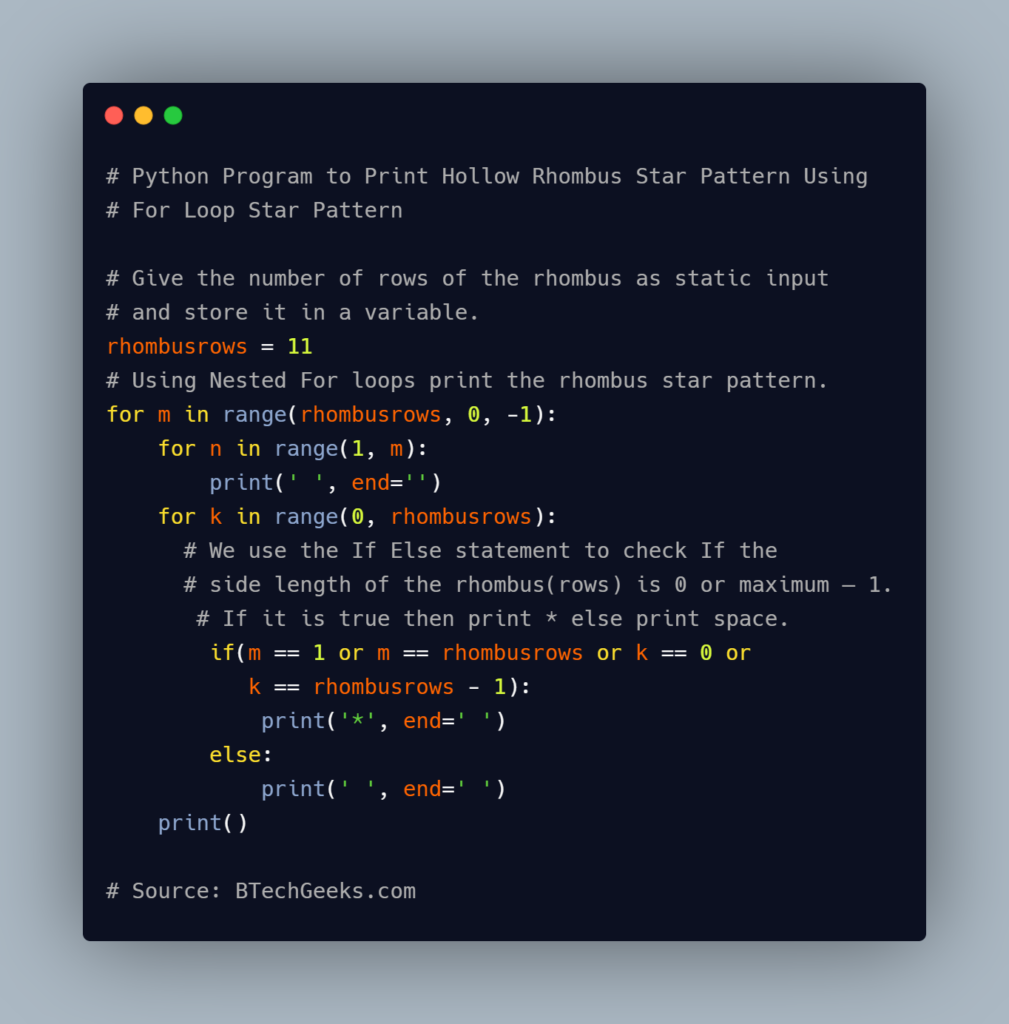# Python Program to Print Hollow Rhombus Star Pattern

Grab the opportunity to learn all effective java programming language concepts from basic to advance levels by practicing these Java Program Examples with Output

Given the number of rows of the rhombus, the task is to print the hollow rhombus Star Pattern in C, C++, and Python.

Examples:

Example1:

Input:

given number of rows of rhombus =11

Output:

          * * * * * * * * * * *
*                        *
*                        *
*                        *
*                        *
*                        *
*                        *
*                        *
*                        *
*                        *
* * * * * * * * * * *

Example2:

Input:

given number of rows of rhombus =6
given character to print =<

Output:

          < < < < < <
<               <
<               <
<               <
<               <
< < < < < <

## Program to Print Hollow Rhombus Star Pattern in C, C++, and Python

Below are the ways to print Hollow Rhombus star patterns in C, C++, and Python.

### Method #1: Using For Loop (Star Character)

Approach:

• Give the number of rows of the rhombus as static input and store it in a variable.
• Using Nested For loops print the rhombus star pattern.
• We use the If Else statement to check If the side length of the rhombus(rows) is 0 or maximum – 1.
• If it is true then print * else print space.
• The Exit of the Program.

1) Python Implementation

Below is the implementation: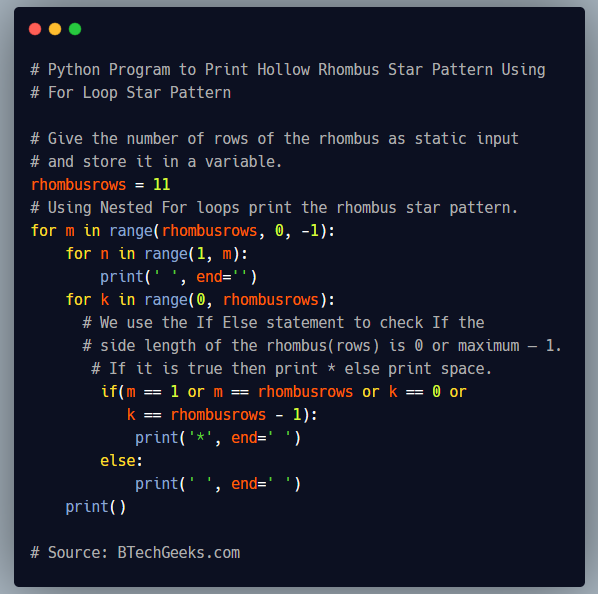# Give the number of rows of the rhombus as static input and store it in a variable.
rhombusrows = 11
# Using Nested For loops print the rhombus star pattern.
for m in range(rhombusrows, 0, -1):
for n in range(1, m):
print(' ', end='')
for k in range(0, rhombusrows):
# We use the If Else statement to check If the side length of the rhombus(rows) is 0 or maximum – 1.
# If it is true then print * else print space.
if(m == 1 or m == rhombusrows or k == 0 or k == rhombusrows - 1):
print('*', end=' ')
else:
print(' ', end=' ')
print()


Output:

          * * * * * * * * * * *
*                        *
*                        *
*                        *
*                        *
*                        *
*                        *
*                        *
*                        *
*                        *
* * * * * * * * * * *

2) C++Implementation

Below is the implementation: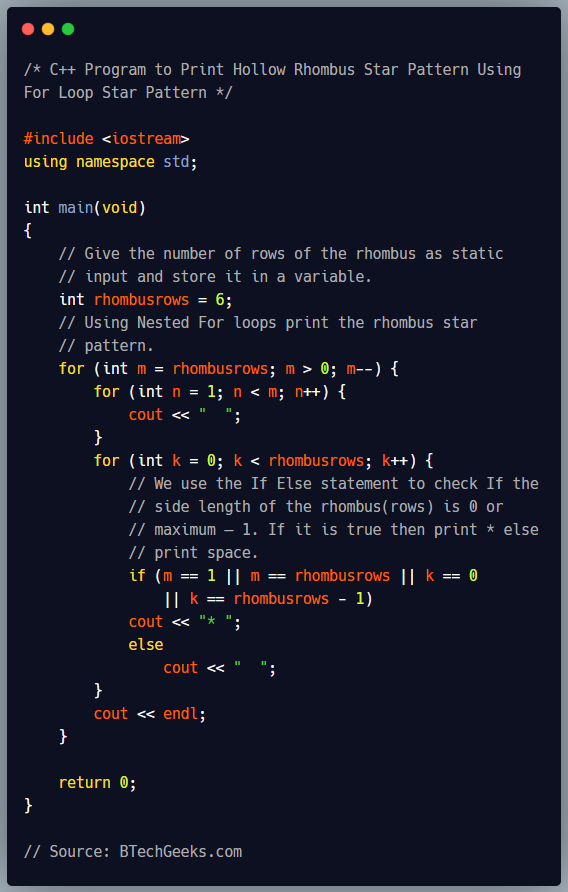#include <iostream>
using namespace std;

int main(void)
{
// Give the number of rows of the rhombus as static
// input and store it in a variable.
int rhombusrows = 6;
// Using Nested For loops print the rhombus star
// pattern.
for (int m = rhombusrows; m > 0; m--) {
for (int n = 1; n < m; n++) {
cout << "  ";
}
for (int k = 0; k < rhombusrows; k++) {
// We use the If Else statement to check If the
// side length of the rhombus(rows) is 0 or
// maximum – 1. If it is true then print * else
// print space.
if (m == 1 || m == rhombusrows || k == 0
|| k == rhombusrows - 1)
cout << "* ";
else
cout << "  ";
}
cout << endl;
}

return 0;
}

Output:

          * * * * * *
*           *
*           *
*           *
*           *
* * * * * *

3) C Implementation

Below is the implementation: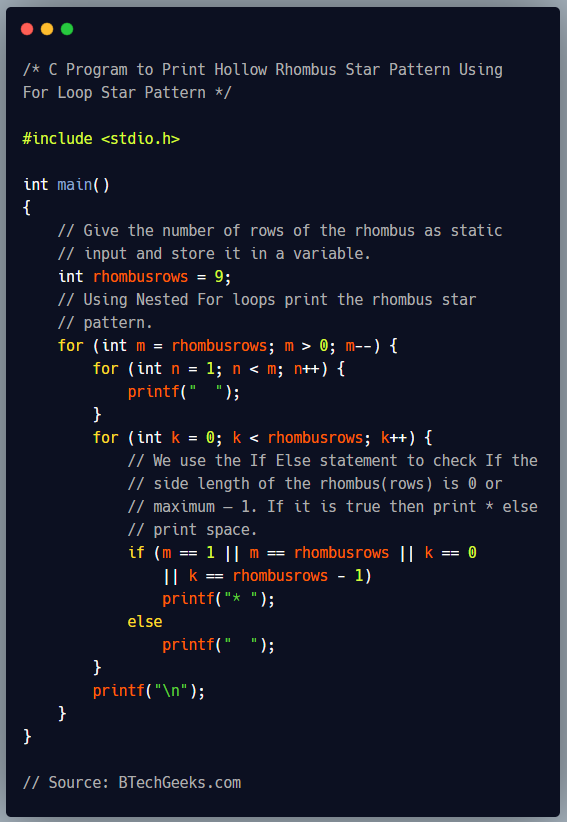#include <stdio.h>

int main()
{
// Give the number of rows of the rhombus as static
// input and store it in a variable.
int rhombusrows = 9;
// Using Nested For loops print the rhombus star
// pattern.
for (int m = rhombusrows; m > 0; m--) {
for (int n = 1; n < m; n++) {
printf("  ");
}
for (int k = 0; k < rhombusrows; k++) {
// We use the If Else statement to check If the
// side length of the rhombus(rows) is 0 or
// maximum – 1. If it is true then print * else
// print space.
if (m == 1 || m == rhombusrows || k == 0
|| k == rhombusrows - 1)
printf("* ");
else
printf("  ");
}
printf("\n");
}
}

Output:

                * * * * * * * * *
*                   *
*                   *
*                   *
*                   *
*                   *
*                   *
*                   *
* * * * * * * * *

### Method #2: Using For Loop (User Character)

Approach:

• Give the number of rows of the rhombus as static input and store it in a variable.
• Scan the character to print as user input and store it in a variable.
• Using Nested For loops print the rhombus star pattern.
• We use the If Else statement to check If the side length of the rhombus(rows) is 0 or maximum – 1.
• If it is true then print * else print space.
• The Exit of the Program.

1) Python Implementation

• Give the number of sides of the rhombus as user input using int(input()) and store it in a variable.
• Give the Character as user input using input() and store it in another variable.

Below is the implementation: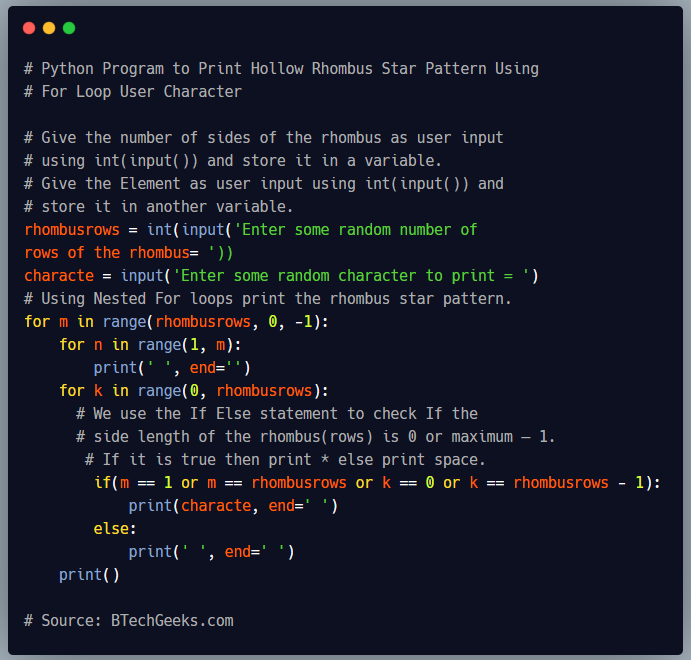# Give the number of sides of the rhombus as user input using int(input()) and store it in a variable.
# Give the Element as user input using int(input()) and store it in another variable.
rhombusrows = int(input('Enter some random number of rows of the rhombus= '))
characte = input('Enter some random character to print = ')
# Using Nested For loops print the rhombus star pattern.
for m in range(rhombusrows, 0, -1):
for n in range(1, m):
print(' ', end='')
for k in range(0, rhombusrows):
# We use the If Else statement to check If the side length of the rhombus(rows) is 0 or maximum – 1.
# If it is true then print * else print space.
if(m == 1 or m == rhombusrows or k == 0 or k == rhombusrows - 1):
print(characte, end=' ')
else:
print(' ', end=' ')
print()


Output:

Enter some random number of rows of the rhombus= 6
Enter some random character to print = <
< < < < < <
<               <
<               <
<               <
<               <
< < < < < <

2) C++Implementation

• Give the number of sides of the rhombus as user input using cin and store it in a variable.
• Create a character variable.
• Give the character as user input using cin and store it in another variable.

Below is the implementation: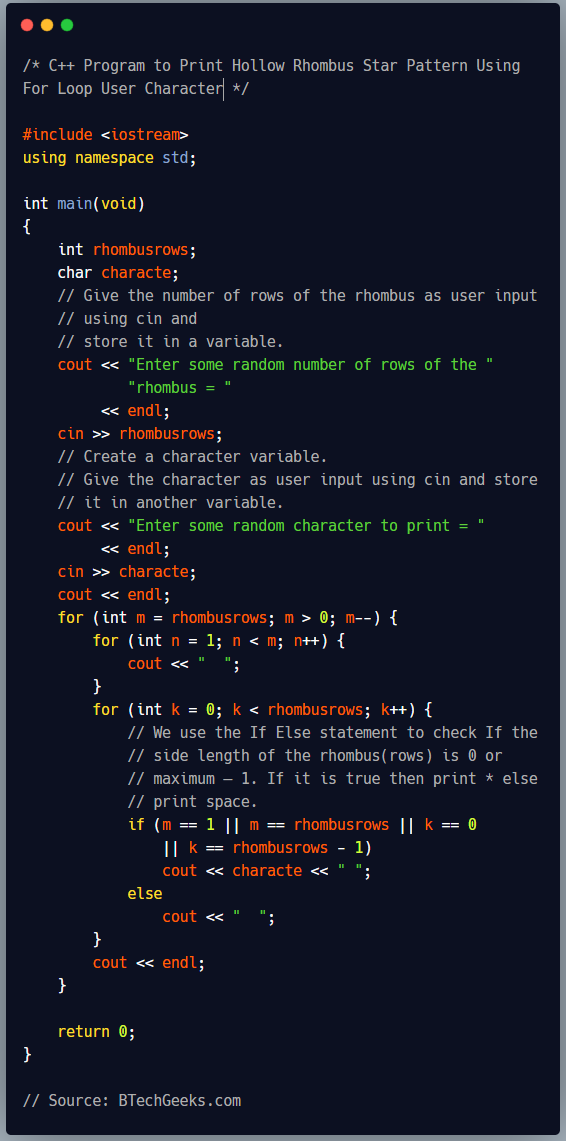#include <iostream>
using namespace std;

int main(void)
{
int rhombusrows;
char characte;
// Give the number of rows of the rhombus as user input
// using cin and
// store it in a variable.
cout << "Enter some random number of rows of the "
"rhombus = "
<< endl;
cin >> rhombusrows;
// Create a character variable.
// Give the character as user input using cin and store
// it in another variable.
cout << "Enter some random character to print = "
<< endl;
cin >> characte;
cout << endl;
for (int m = rhombusrows; m > 0; m--) {
for (int n = 1; n < m; n++) {
cout << "  ";
}
for (int k = 0; k < rhombusrows; k++) {
// We use the If Else statement to check If the
// side length of the rhombus(rows) is 0 or
// maximum – 1. If it is true then print * else
// print space.
if (m == 1 || m == rhombusrows || k == 0
|| k == rhombusrows - 1)
cout << characte << " ";
else
cout << "  ";
}
cout << endl;
}

return 0;
}

Output:

Enter some random number of rows of the rhombus =
5
Enter some random character to print =
?
? ? ? ? ?
?         ?
?         ?
?         ?
? ? ? ? ?

3) C Implementation

• Give the number of sides of the rhombus as user input using scanf and store it in a variable.
• Create a character variable.
• Give the character as user input using scanf and store it in another variable.

Below is the implementation: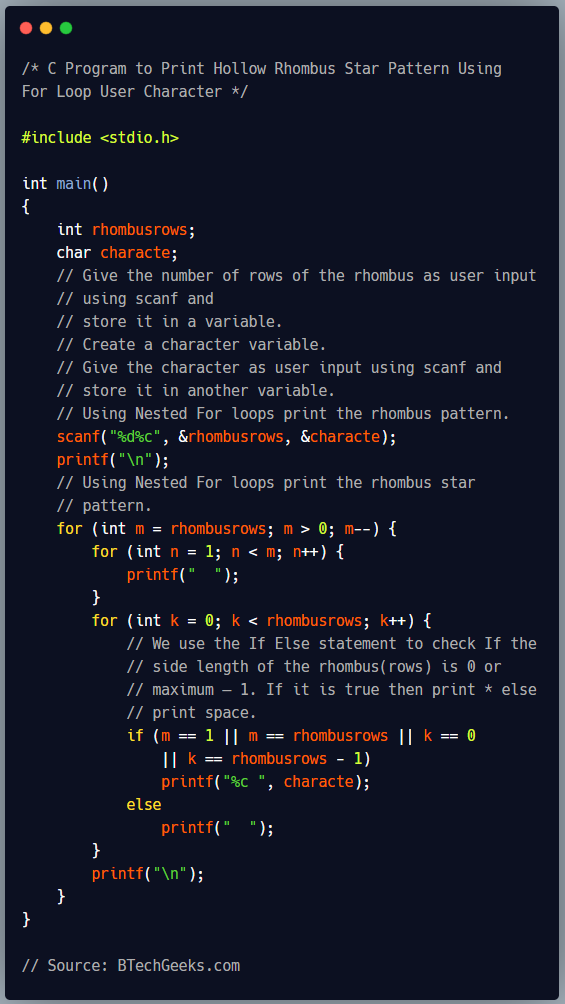#include <stdio.h>

int main()
{
int rhombusrows;
char characte;
// Give the number of rows of the rhombus as user input
// using scanf and
// store it in a variable.
// Create a character variable.
// Give the character as user input using scanf and
// store it in another variable.
// Using Nested For loops print the rhombus pattern.
scanf("%d%c", &rhombusrows, &characte);
printf("\n");
// Using Nested For loops print the rhombus star
// pattern.
for (int m = rhombusrows; m > 0; m--) {
for (int n = 1; n < m; n++) {
printf("  ");
}
for (int k = 0; k < rhombusrows; k++) {
// We use the If Else statement to check If the
// side length of the rhombus(rows) is 0 or
// maximum – 1. If it is true then print * else
// print space.
if (m == 1 || m == rhombusrows || k == 0
|| k == rhombusrows - 1)
printf("%c ", characte);
else
printf("  ");
}
printf("\n");
}
}

Output:

5 :
: : : : :
:       :
:       :
:       :
: : : : :

Related Programs: